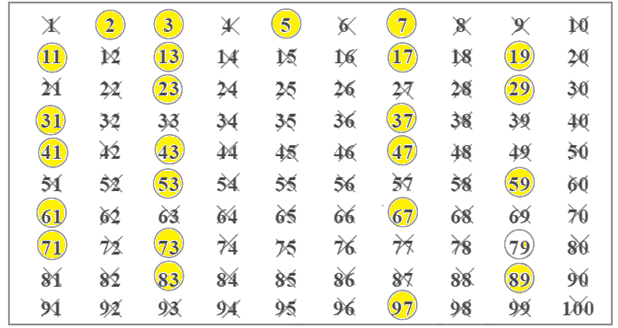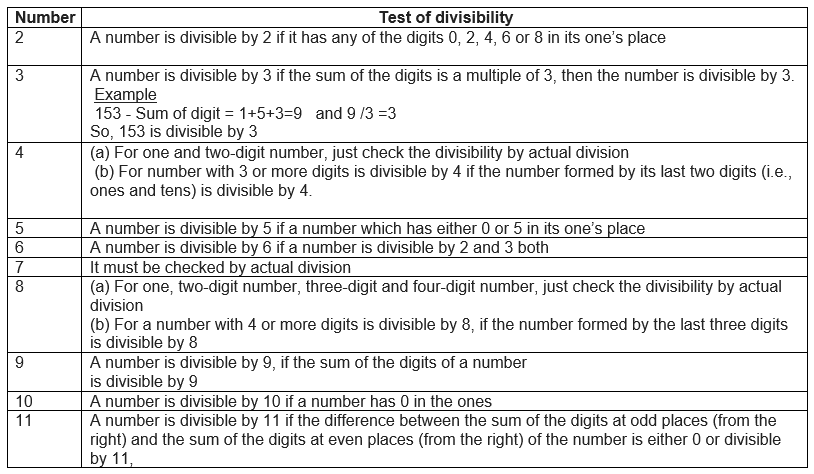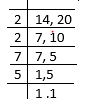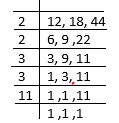# Playing With Numbers Class 6 Notes

These are of chapter 3 Playing with Numbers class 6 notes. Hope you like these Playing with Numbers notes and share the page among your friends.
Topics of Divisibility Rules based on factors and Multiple, co -prime is deleted as per Rationalized Syllabus
Table of Content

## What are Factors?

A factor of a number is an exact divisor of that number
Example
1. Factor of 6
1-> Since 1 exactly divides 6
2 -> Since it exactly divides 6
3 -> Since it exactly divides 6
6-> Since it exactly divides 6

### Properties of factors

1. 1 is a factor of every number
2. every number is a factor of itself.
3. every factor of a number is an exact divisor of that number
4. every factor is less than or equal to the given number
5. number of factors of a given number are finite.

## Multiple

Multiple of a number is the numbers obtained by multiplying that numbers with various Natural numbers
Example
Number is 6
Multiple will be
$6 \times 1= 6$
$6 \times 2=12$
$6 \times 3=18$

### Properties of Multiple

1. Every multiple of a number is greater than or equal to that number.
2. number of multiples of a given number is infinite
3. every number is a multiple of itself

## Perfect Number

A number for which sum of all its factors is equal to twice the number is called a perfect number
Example
1. 6
The factors of 6 are 1, 2, 3 and 6.
Now, $1+2+3+6 = 12 = 2 \times 6$.
2. 28
All the factors of 28 are 1, 2, 4, 7, 14 and 28.
Now, $1 + 2 + 4 + 7 + 14 + 28 = 56 = 2 \times 28$.

## Prime Numbers

The numbers other than 1 whose only factors are 1 and the number itself are called Prime numbers.
Example:
2, 3, 5, 7, 11 ,13
We can find list of prime numbers till 100 using Sieve of Eratosthenes methodStep 1: Cross out 1 because it is not a prime number.
Step 2: Encircle 2, cross out all the multiples of 2, other than 2 itself, i.e. 4, 6, 8 and so on.
Step 3: You will find that the next uncrossed number is 3. Encircle 3 and cross out all the multiples of 3, other than 3 itself.
Step 4: The next uncrossed number is 5. Encircle 5 and cross out all the multiples of 5 other than 5 itself.
Step 5: Continue this process till all the numbers in the list are either encircled or crossed out.
All the encircled numbers are prime numbers. All the crossed-out numbers, other than 1 are composite numbers

## Composite Numbers

Numbers having more than two factors (1 and itself) are called Composite numbers
Example:
4, 6, 8 ,9….

## Even Numbers

The numbers which are multiple of 2 are called even numbers
Example
2,4,6,8,10,12,14
Even numbers have 0,2,4,6,8 in it one’s place

## Odd Numbers

The numbers which are not multiple of 2 are called odd numbers
Example
1,3, 5,7,9,11......
Important points about prime numbers based on definition of odd and even numbers
1. 2 is the smallest prime number which is even.
2. every prime number except 2 is odd.

## Tests for Divisibility of Numbers## Common Factors and Multiple

We already studied the factors and Multiple in previous section.  We can find common factors and multiple between 2 or more numbers
Example
1) Common factors of 4,12, and 16
Factors of 4 are 1, 2 and 4.
Factors of 12 are 1, 2, 3, 4, 6 and 12.
Factors of 16 are 1, 2, 4, 8 and 16.
Clearly, 1, 2 and 4 are the common factors of 4, 12, and 16.
Important Note
Two numbers having only 1 as a common factor are called co-prime number
Example   3 and 4 are co-prime

## Divisibility Rules based on factors and Multiple, co -prime

1) if a number is divisible by another number then it is divisible by each of the factors of that number
Example
36, is divisible by 18
Now if we find factors of 18 i.e., 1,2 ,3,4,9,18
So, 36 is also divisible by 1,2 ,3,4,9,18
2) If a number is divisible by two co-prime numbers then it is divisible by their product also
Example
45
It is divisible by 3 (4+5=9)
It is divisible by 5
Since 3,5 are coprime. Now the product is 3 X5=15. Now it is divisible by 15 also
3) If two given numbers are divisible by a number, then their sum is also divisible by that number.
Example
15 is divisible by 3
9 is divisible by 3
Sum = 15+9 =24
We can see that it is also divisible by 3
4) If two given numbers are divisible by a number, then their difference is also divisible by that number
Example
15 is divisible by 3
9 is divisible by 3
Difference = 15 - 9 =6
We can see that it is also divisible by 3

## Factorisation and Prime -Factorisation

Factorisation is expressing the number as a product of its factors
So,
36 = 3×12 = 4 X 9
These form is called Factorisation
Prime Factorisation is expressing the number as a product of its prime factors
$36 = 2 \times 2 \times 3 \times 3$
We can find prime factorisation by dividing the numbers with 2, 3, 5, 7 etc. in this order repeatedly so long as the quotient is divisible by that number

## HCF and LCM

a. The Highest Common Factor (HCF) of two or more given numbers is the highest of their common factors. It is also known as Greatest Common Divisor (GCD).
Steps to find HCF or GCD
a. Find the prime factorisation of the numbers
b. Choose the common factors in them
c. Multiply those common factors to obtain HCF

Example
(a) Find the HCF of 8 and 12
Prime Factorisation of the numbers
$8 = 2 \times 2 \times 2$
$12 = 2 \times 2 \times 3$
Common factors are 2,2
So $HCF = 2 \times 2 =4$
(b) Find the HCF of 20, 28 and 36
Prime Factorisation of the numbers
$20 = 2 \times 2 \times 5$
$28 = 2 \times 2 \times 7$
$36=2 \times 2 \times 3 \times 3$
Common factors are 2,2
So $HCF = 2 \times 2 =4$

(b) The Lowest Common Multiple (LCM) of two or more given numbers is the lowest of their common multiples.
Steps to find LCM
(a) Find the prime factorisation of the numbers
(b) look for the maximum occurrence of all the prime factors in these numbers
(c) The LCM of the numbers will be the product of the prime factors counted the maximum number of times they occur in any of the numbers

Example
(a) Find the LCM of 8 and 12
Prime Factorisation of the numbers
$8 = 2 \times 2 \times 2$
$12 = 2 \times 2 \times 3$
So, $LCM = (2 \times 2 \times 2) \times (3) = 24$
(b) Find the LCM of 20, 28 and 36
Prime Factorisation of the numbers
$20 = 2 \times 2 \times 5$
$28 = 2 \times 2 \times 7$
$36=2 \times 2 \times 3 \times 3$
$LCM = (2 \times 2) \times (3) \times (5) \times (7) =540$

LCM using division method
Here we divide the given numbers by common prime number until the remainder is a prime number or one. LCM will be the product obtained by multiplying all divisors and remaining prime numbers.
Steps are
(1) We place number in the line
(2) We start dividing the number by least prime number which is common among all of them or group of them
(3) Keep dividing by least until we have 1's in the remainder
(4) LCM is the product of the divisors

Example
(1) Find the LCM of 14 and 20$LCM = 2 \times 2 \times 7 \times 5=140$
(2) Find the LCM of 12 ,18 and 44$LCM = 2 \times 2 \times 3 \times 3 \times 11=396$### Practice Question

Question 1 What is $\frac {1}{2} + \frac {3}{4}$ ?
A)$\frac {5}{4}$
B)$\frac {1}{4}$
C)$1$
D)$\frac {4}{5}$
Question 2 Pinhole camera produces an ?
A)An erect and small image
B)an Inverted and small image
C)An inverted and enlarged image
D)None of the above